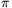Mathematical and Physical Journal
for High Schools
Issued by the MATFUND Foundation
 Already signed up? New to KöMaL?

#Problem C. 886. (February 2007)

C. 886. The wall of a building is decorated by a square with its circumscribed circle drawn. There is a semicircle drawn outwards on each side of the square as a diameter, so that the circular arcs form four crescent shaped figures. What is the length of the side of the square if the area of such a crescent is 1 m2?

(5 pont)

Deadline expired on March 19, 2007.

Sorry, the solution is available only in Hungarian. Google translation

Megoldás. Jelölje a négyzet oldalát x. Ekkor egy Hold alakú mező területe:=x2(/8-/8+1/4)=x2/4.

Vagyis 1=x2/4, ahonnan x=2 m.

### Statistics:

 410 students sent a solution. 5 points: 357 students. 4 points: 8 students. 3 points: 35 students. 2 points: 7 students. 0 point: 2 students. Unfair, not evaluated: 1 solutions.

Problems in Mathematics of KöMaL, February 2007